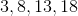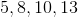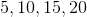# High School Math : Summations and Sequences

## Example Questions

### Example Question #1 : Summations And Sequences

Consider the Fibonacci sequence written below. What are the next three terms?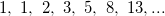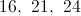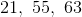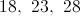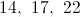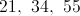Explanation:

Each number in the Fibonacci sequence can be calculated by the sum of the previous two numbers.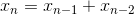The next term in the sequence will be the sum of the two terms preceding it: 8 and 13.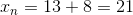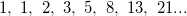The next term will be the sum of 13 and 21.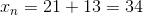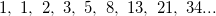The next term will be the sum of 21 and 34.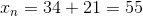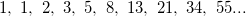### Example Question #1 : Geometric Sequences

Which of the following is a geometric sequence?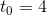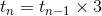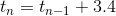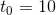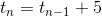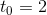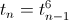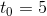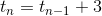Explanation:

A geometric sequence is one in which the next term is found by mutlplying the previous term by a particular constant. Thus, we look for an implicit definition which involves multiplication of the previous term. The only possibility is:### Example Question #1 : Arithmetic Series

List the first 4 terms of an arithmetic sequence with a first term of 3, and a common difference of 5.